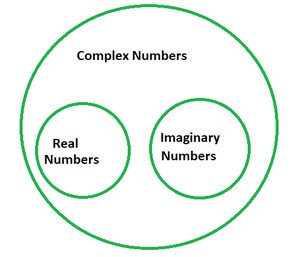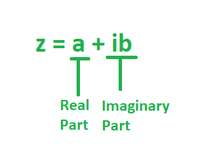GeeksforGeeks App
Open AppBrowser
Continue

# Decompose f(z) = z2 into its real and imaginary parts

Everyone is familiar with the concept of equations in mathematics and their various types such as, (x + p = q); (x × p = q), (x2 = a), etc. But what would one be doing if the given equation is x2 + 69 = 0? How to solve this? Following basic arithmetic operations are derived: x =. Is this even possible? Yes, with the concept of complex numbers, equations like these can be solved with feasible solutions.

Complex Numbers

Complex numbers are those numbers that are made up of both real and imaginary constituents and can be expressed in the form of z = a + ib, where i (iota) = √(-1), a and b represent real and imaginary parts respectively. For example, 69 + 420i is a complex number where 69 is a real number and 420i is its imaginary constituent.### Identifying Real and Imaginary Parts

In simple words, the part of a complex number that is written without iota(i) is called the real part and the one which is accompanied with iota is called the imaginary part. This is the case employed for the complex numbers which are already in their rectangular/ standard form. In case a quadratic, cubic, or some other expression is given, it first should be evaluated then the real and imaginary parts be determined.For example, (1 + i)2 needs to be expanded first using the identity (a + b)2 = a2 + 2ab + b2, then be decomposed into real and imaginary components.

### Decompose f(z) = z2 into its real and imaginary parts.

Solution:

Given: w = f(z) = z2

Let z = x + iy, as per the standard form of a complex number.

⇒ z2 = (x + iy)2 = x2 + 2(x)(iy) + (iy)2

= x2 – y2 + i(2xy)

Hence, the real part is x2 – y2 and the imaginary part is 2xy.

### Similar Problems

Question 1: Find the real and imaginary parts of (1 + i)2.

Solution:

(1 + i)2 = 12 + i2 + 2i

= 1 – 1 + 2i

= 2i

Thus, (1 + i)2 has no real part and the imaginary part is 2.

Question 2: Find the real and imaginary parts of (1 – i)2.

Solution:

(1 – i)2 = 12 + i2 – 2i

= 1 – 1 – 2i

= -2i

The real part of (1 – i)2 is 0 and the imaginary part is -2i.

Question 3: Find the real and imaginary parts of (3 + 2i)2.

Solution:

(3 + 2i)2 = 32 + 2(2i)(3) + (2i)2

= 9 + 4(-1) + 12i

= 5 + 12i

Thus, real part is 5 and the imaginary part is 12.

Question 4: Find the real and imaginary parts of (12 + 6i)2.

Solution:

(12 + 6i)2 = 122 + 2(12)(6i) + (6i)2

= 144 + 36(-1) + 144i

= 108 + 144i

Thus, real part is 108 and the imaginary part is 144.

Question 5: Find the real and imaginary parts of (10 + 10i)2.

Solution:

(10 + 10i)2 = 102 + 2(10)(10i) + (10i)2

= 100 + 200(-1) + 100i

= -100 + 100i

Thus, real part is -100 and the imaginary part is 100.

My Personal Notes arrow_drop_up
Related Tutorials